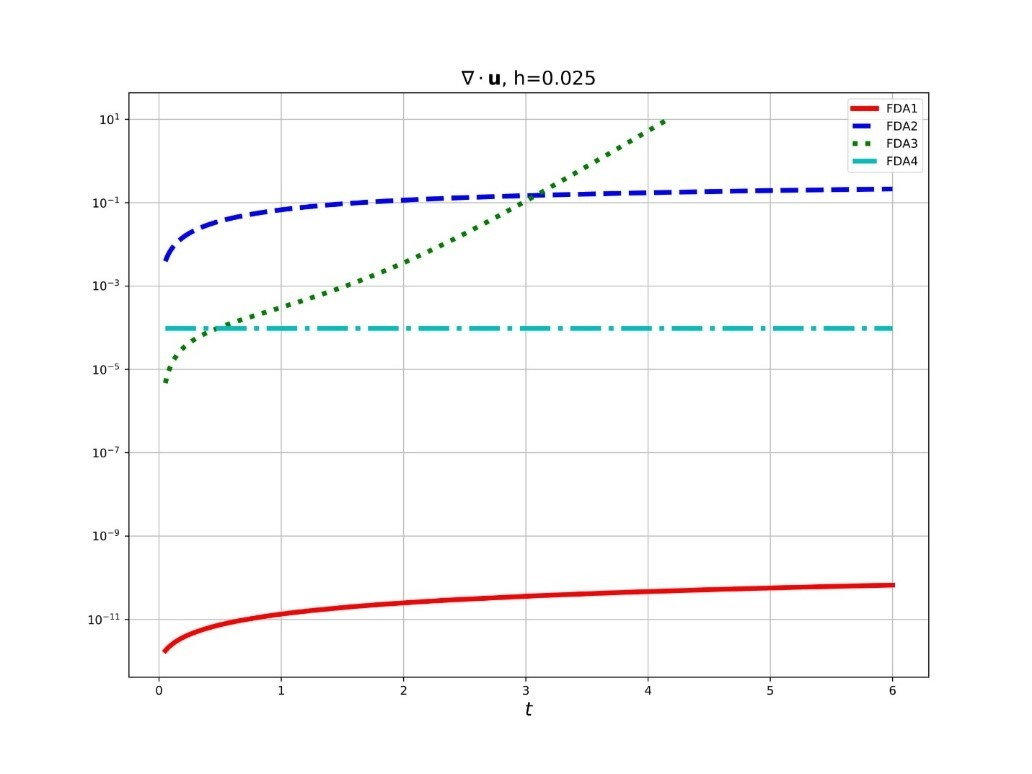The challenge of the Millennium. One step closer to the solution

# The challenge of the Millennium. One step closer to the solution

In 2017, RUDN University scientists constructed a new explicit second-order precision difference scheme using modern computer algebra methods for 2-dimensional Navier-Stokes equations (NSE) . This year, our mathematicians used a new scheme  to construct a numerical solution to the Cauchy problem with initial data (for t=0) as satisfying the continuity equation. Scientists managed to achieve previously unattainable accuracy of the continuity equation.The main purpose of the Navier-Stokes equations is to show how mathematics can be applied in practice in solving problems of modeling liquids and gases. For example, when modeling weather, ocean currents, air flow in a pipe or around an airplane wing. Since the 19th century, these equations have been used to describe the phenomena of the physical world. RUDN University scientists managed to significantly improve the quality of approximation of the numerical solution of a system of differential equations.

The Navier-Stokes equations (NSE) are a system of nonlinear partial differential equations. These are the most important equations in hydrodynamics: they are used for mathematical modeling of many natural phenomena and technical devices.

In the case of a mathematical model of a continuous medium-a viscous incompressible liquid, for example, water, the system includes an incompressibility equation, usually called the continuity equation. This is a linear partial differential equation, which is a mathematical formulation of the law of conservation of the total mass of the liquid involved in the process under study. The remaining equations of the NSE system are nonlinear equations and are a mathematical formulation of the law of conservation of momentum for the elements of the volume of a liquid during its flow. From these equations, it is convenient to find by numerical methods the distribution of flow velocities in the considered volume of liquid. Depending on the process under study, the fluid flow can be considered as one-dimensional, two-dimensional or three-dimensional. This means that the variables describing fluid dynamics, i.e. the spatial components of velocity and pressure, depend, respectively, on one, two or three spatial coordinates. In addition, for a non-stationary (unsteady) flow, all these variables also depend on time.

The analysis of finite-energy NSE solutions, namely, the rigorous proof of the existence of such solutions, is the essence of one of the six unsolved “Millennium problems” for which the clay Mathematical Institute has awarded a \$ 1 million prize. It is necessary to prove or disprove the existence of a global error-free solution of the Cauchy problem for three-dimensional NSE, which has finite energy.

A General analytical solution of the NSE, both for spatial and plane flow, cannot be found due to non-linearity and strong dependence on initial and/or boundary conditions. It remains to solve the NSE by numerical methods, for which the differential equations must be approximated, i.e. consciously simplified, by discrete equations. But at the same time, the challenge remains the construction of such an approximation, which inherits at a discrete level, with a sufficiently high accuracy, not only all the conservation laws (momentum and mass) inherent in the UNS, but also all the mathematical consequences of these equations.

In their 2017 paper , our scientists used modern computer algebra to construct a new second-order precision difference scheme for 2-dimensional NSE. The scheme on the test example of the only known non-stationary exact solution of the NSE showed very good numerical behavior.

New numerical results obtained in the 2020 paper “Strong Consistency and Thomas Decomposition of Finite Difference Approximations to Systems of Partial Differential Equations” are shown in the figure below, where FDA1 is the described scheme and FDA2-FDA4 are the other schemes used for comparison. From the figure taken from the paper, it can be seen that the continuity equation is performed by the FDA1 scheme with an accuracy of 6 or more orders of magnitude higher than the accuracy of this equation by other explicit second-order schemes.

This accuracy of the continuity equation without its explicit consideration in solving the Cauchy problem is achieved for the first time and is an unprecedented result. In addition, the fda1 scheme is significantly superior (by 10 or more times) to the FDA2-FDA4 schemes, both in terms of the accuracy of calculating speeds and pressure.

Millennium problems — in 2000, the Clay Mathematical Institute announced a list of the most important classical problems that have remained unanswered for many years. For solving each of the problems, now there are six of them, the clay Institute has appointed a prize of 1,000,000 US dollars. One of the Millennium problems (the Poincare hypothesis) was solved by the Russian mathematician G.Ya. Perelman in 2002-2003.

The Clay Mathematical Institute is a private Institute in the United States, founded in 1998. The main goal of the mathematical Institute is to increase and disseminate knowledge in the field of mathematics.

The Cauchy problem is one of the main problems of the theory of differential equations. It consists in finding a solution (integral) of a differential equation that satisfies the so-called initial conditions (initial data).

Visiting Professors View all
03 Nov 2017
Michele Pagano is a graduate of the University of Pisa, a leading scientist, the author of more than 200 publications in international journals, and a participant in many international research projects
2573
International Projects View all
15 Dec 2018
1171
07 Jul
RUDN University Biologist: salted water saves fish from stress when transporting

RUDN University biologist with colleagues from Brazil and Iran found how to alleviate the stress of fish during transportation. It turned out that this can be achieved with salted water.

62
20 Apr
RUDN University agronomists increased wheat yield by 65%

RUDN University agronomists have proposed a new scheme for fertilizing winter wheat, which allows increasing the yield by 68%. The key to this is in the combination of nitrogen and growth regulators.

123
20 Apr
RUDN Biologists Study Live Microorganisms in Toxic Liquids for Metalworking

RUDN biologists have studied microorganisms that can survive in metalworking fluids. The results will allow “picking up” bacteria and fungi that can process toxic waste fluids into a harmless product.

117